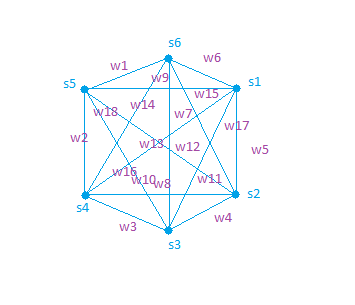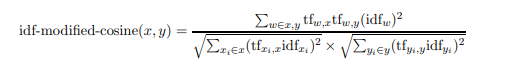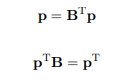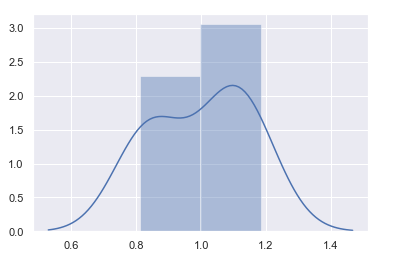×

Search anything:

# LexRank method for Text Summarization

#### Machine Learning (ML) Natural Language Processing (NLP)Get this book -> Problems on Array: For Interviews and Competitive Programming

Reading time: 35 minutes | Coding time: 15 minutes

LexRank method for text summarization is another child method to PageRank method with a sibling TextRank. It uses a graph based approach for automatic text summarization. In this article we will try to learn the concept of LexRank and various methods to implement the same in Python.

## Introduction

LexRank is an unsupervised graph based approach for automatic text summarization. The scoring of sentences is done using the graph method. LexRank is used for computing sentence importance based on the concept of eigenvector centrality in a graph representation of sentences.

In this model,we have a connectivity matrix based on intra-sentence cosine similarity which is used as the adjacency matrix of the graph representation of sentences. This sentence extraction majorly revolves around the set of sentences with same intend i.e. a centroid sentence is selected which works as the mean for all other sentences in the document. Then the sentences are ranked according to their similarities.

Target Document:- Any Multi-set documents.

### Graphical Approach

1. Based on Eigen Vector Centrality.
2. Sentences are placed at the vertexes of the Graphs
3. The weight on the Edges are calculated using cosine similarity metric.Where Si are the sentences at the vertices respectively and Wij are weights on the edges.

## Cosine similarity Computation

I order to Define similarity ,bag of words model is used to represent N-dimensional vectors where N is the number of all possible words in words in a particular language. For each word that occurs in a sentence, the value of the corresponding dimension in the vector representation of the sentence is the number of occurrences of the word in the sentence times the idf of the word.Where tfw,s is the number of occurrences of the word w in the sentence s.

## Eigen Vector Centrality and LexRank

Each nodes contribution is calculated to determine the overall centrality. But it is not necessary that in each graphical-network all the Nodes are considered equally important. If there are unrelated documents with significantly important sentences then these sentences will get high centrality scores automatically. This situation is avoided by considering the origin nodes. Thus a simple method to calculate centrality is :Where matrix B is the adjancy matrix obtained from the similarity graph by dividing each element by the corresponding row sum. pT is the left eigenvector of the matrix B with the corresponding eigenvalue of 1.

## Algorithm

Algortihm to calculate LexRank Scores is as follows:

``````1.MInputAn array S of n sentences, cosine threshold t output: An array L of LexRank scores
2.Array CosineMatrix[n][n];
3.Array Degree[n];
4.Array L[n];
5.for i ← 1 to n do
6.     for j ← 1 to n do
7.          CosineMatrix[i][j] = idf-modified-cosine(S[i],S[j]);
8.          if CosineMatrix[i][j] > t then
9.               CosineMatrix[i][j] = 1;
10.               Degree[i] + +;
11.          end
12.          else
13.               CosineMatrix[i][j] = 0;
14.          end
15.     end
16.end
17.for i ← 1 to n do
18.     for j ← 1 to n do
19.          CosineMatrix[i][j] = CosineMatrix[i][j]/Degree[i];
20.     end
21.end
22. L = PowerMethod(CosineMatrix,n,ǫ);
23. return L;
``````

In the first 4 steps we initialize the required arrays for sentences, threshold, LexRank Scores, Cosine matrix, Degree and Eigen Values respectively.

The steps 5 to 9, initialize our matrix by tf-idf modified values for the given sentences. If the values are greater than a thershold idf value then the element is replaced by value 1. If it is less than the threshold required than the value is replaced by 0. Thus we create a typical tf-idf table or matrix in these steps.
In step 17 to 21 each value of cosine matrix is divided by degree of each node. Degree centrality here is the corresponding degree of each node.

Finally through the Power iteration method we calculate the final score.

## Power Iteration method

Power Iteration or Power method is basically used to calculate the value of largest eigen vector of a matrix. For a diagonalizable matrix A, the algorithm will produce a number lambda , which is the greatest (in absolute value) eigenvalue of A, and a nonzero vector v, which is a corresponding eigenvector of lambda , that is, Av=lambda v.

Computations-

The method has the recurrence relationWhere bo is the approximation to dominant eigen vector. So, at every iteration, the vector b is multiplied by the matrix A and normalized.

Complexity= O(n2)

## Differences Between LexRank and TextRank

There is a very minute margin of differences between the both:

S.no. LexRank TextRank
1 In addition to pageRank approach, it uses similarity metrics Uses typical PageRank approach
2 Considers position and length of sentences Does not consider any such parameters
3 Use for Multi-document summarization Used for Single document summarization

# Implementation

## PseudoCode

Defining the basic properties of LexRank summarizer i.e. defining threshold and epsilon values. The other characteristics include stop word removal,tf-idf transformations and matrix formation for score calculations and cosine similarity models. All these models are described by the code as follows:

``````class LexRankSummarizer():
threshold = 0.1
epsilon = 0.1
_stop_words = frozenset()
@property
def stop_words(self):
return self._stop_words

@stop_words.setter
def stop_words(self, words):
self._stop_words = frozenset(map(self.normalize_word, words))
``````

Using LexRank on a set of Sentences using Tf-idf metrics

``````    def __call__(self, document, sentences_count):
self._ensure_dependencies_installed()

sentences_words = [self._to_words_set(s) for s in document.sentences]
if not sentences_words:
return tuple()

tf_metrics = self._compute_tf(sentences_words)
idf_metrics = self._compute_idf(sentences_words)

matrix = self._create_matrix(sentences_words, self.threshold, tf_metrics, idf_metrics)
scores = self.power_method(matrix, self.epsilon)
ratings = dict(zip(document.sentences, scores))

return self._get_best_sentences(document.sentences, sentences_count, ratings)
``````

Computing the tf values

``````    def _compute_tf(self, sentences):
tf_values = map(Counter, sentences)

tf_metrics = []
for sentence in tf_values:
metrics = {}
max_tf = self._find_tf_max(sentence)

for term, tf in sentence.items():
metrics[term] = tf / max_tf

tf_metrics.append(metrics)

return tf_metrics
``````

Creating the matrices

``````     def _create_matrix(self, sentences, threshold, tf_metrics, idf_metrics):
"""
Creates matrix of shape |sentences|×|sentences|.
"""
# create matrix |sentences|×|sentences| filled with zeroes
sentences_count = len(sentences)
matrix = numpy.zeros((sentences_count, sentences_count))
degrees = numpy.zeros((sentences_count, ))

for row, (sentence1, tf1) in enumerate(zip(sentences, tf_metrics)):
for col, (sentence2, tf2) in enumerate(zip(sentences, tf_metrics)):
matrix[row, col] = self.cosine_similarity(sentence1, sentence2, tf1, tf2, idf_metrics)

if matrix[row, col] > threshold:
matrix[row, col] = 1.0
degrees[row] += 1
else:
matrix[row, col] = 0

for row in range(sentences_count):
for col in range(sentences_count):
if degrees[row] == 0:
degrees[row] = 1

matrix[row][col] = matrix[row][col] / degrees[row]

return matrix
``````

Calculating the cosine values and Power values for eigen vectors

``````    @staticmethod
def cosine_similarity(sentence1, sentence2, tf1, tf2, idf_metrics):
"""
We compute idf-modified-cosine(sentence1, sentence2) here.
It's cosine similarity of these two sentences (vectors) A, B computed as cos(x, y) = A . B / (|A| . |B|)
Sentences are represented as vector TF*IDF metrics.
:param sentence1:
Iterable object where every item represents word of 1st sentence.
:param sentence2:
Iterable object where every item represents word of 2nd sentence.
:type tf1: dict
:param tf1:
Term frequencies of words from 1st sentence.
:type tf2: dict
:param tf2:
Term frequencies of words from 2nd sentence
:type idf_metrics: dict
:param idf_metrics:
Inverted document metrics of the sentences. Every sentence is treated as document for this algorithm.
:rtype: float
:return:
Returns -1.0 for opposite similarity, 1.0 for the same sentence and zero for no similarity between sentences.
"""
unique_words1 = frozenset(sentence1)
unique_words2 = frozenset(sentence2)
common_words = unique_words1 & unique_words2

numerator = 0.0
for term in common_words:
numerator += tf1[term]*tf2[term] * idf_metrics[term]**2

denominator1 = sum((tf1[t]*idf_metrics[t])**2 for t in unique_words1)
denominator2 = sum((tf2[t]*idf_metrics[t])**2 for t in unique_words2)

if denominator1 > 0 and denominator2 > 0:
return numerator / (math.sqrt(denominator1) * math.sqrt(denominator2))
else:
return 0.0

@staticmethod
def power_method(matrix, epsilon):
transposed_matrix = matrix.T
sentences_count = len(matrix)
p_vector = numpy.array([1.0 / sentences_count] * sentences_count)
lambda_val = 1.0

while lambda_val > epsilon:
next_p = numpy.dot(transposed_matrix, p_vector)
lambda_val = numpy.linalg.norm(numpy.subtract(next_p, p_vector))
p_vector = next_p

return p_vector
``````

## Implementation using LexRank Library

Python contains a Library LexRank for implementation of LexRank method of summarization. This package is much effective when we have to summarize multiple documents.

Importing the required packages

``````from lexrank import STOPWORDS, LexRank
from path import Path
``````

Setting path to the required set of documents available for summarization

``````documents = []
documents_dir = Path('bbc/politics')

for file_path in documents_dir.files('*.txt'):
with file_path.open(mode='rt', encoding='utf-8') as fp:
``````

Removing stopwords and generating summary based on the sentences provided

``````lxr = LexRank(documents, stopwords=STOPWORDS['en'])

sentences = [
'One of David Cameron\'s closest friends and Conservative allies, '
'George Osborne rose rapidly after becoming MP for Tatton in 2001.',

'Michael Howard promoted him from shadow chief secretary to the '
'Treasury to shadow chancellor in May 2005, at the age of 34.',

'Mr Osborne took a key role in the election campaign and has been at '
'the forefront of the debate on how to deal with the recession and '
'the UK\'s spending deficit.',

'Even before Mr Cameron became leader the two were being likened to '
'Labour\'s Blair/Brown duo. The two have emulated them by becoming '
'prime minister and chancellor, but will want to avoid the spats.',

'Before entering Parliament, he was a special adviser in the '
'agriculture department when the Tories were in government and later '
'served as political secretary to William Hague.',

'The BBC understands that as chancellor, Mr Osborne, along with the '
'Treasury will retain responsibility for overseeing banks and '
'financial regulation.',

'Mr Osborne said the coalition government was planning to change the '
'tax system \"to make it fairer for people on low and middle '
'incomes\", and undertake \"long-term structural reform\" of the '
'banking sector, education and the welfare state.',
]
``````

Generating summary

``````# get summary with classical LexRank algorithm
summary = lxr.get_summary(sentences, summary_size=2, threshold=.1)
print(summary)
``````

Output-

'Mr Osborne said the coalition government was planning to change the tax system "to make it fairer for people on low and middle incomes", and undertake "long-term structural reform" of the banking sector, education and the welfare state.', 'The BBC understands that as chancellor, Mr Osborne, along with the Treasury will retain responsibility for overseeing banks and financial regulation.'

Calculating scores

``````scores_cont = lxr.rank_sentences(
sentences,
threshold=None,
fast_power_method=False,
)
for score in scores_cont:
print(score)
``````

Output-

``````1.0896493024505807
0.9010711968858981
1.113916649701626
0.8279523250808508
0.8112028559566336
1.1852289124853765
1.0709787574388232
``````

Visualization of scores

``````import seaborn as sns
sns.set(color_codes=True)
x = scores_cont
sns.distplot(x)
``````

Output-## Implementation using Sumy Library

Sumy incorporates a quick method for implementation of LexRank technique represented as follows:

Importing the required packages

``````## Using SUmy package
from sumy.parsers.plaintext import PlaintextParser
from sumy.nlp.tokenizers import Tokenizer
from sumy.summarizers.lex_rank import LexRankSummarizer
``````

Representing the text used for summarization

``````doc="""OpenGenus Foundation is an open-source non-profit organization with the aim to enable people to work offline for a longer stretch, reduce the time spent on searching by exploiting the fact that almost 90% of the searches are same for every generation and to make programming more accessible.
OpenGenus is all about positivity and innovation.
Over 1000 people have contributed to our missions and joined our family. We have been sponsored by three great companies namely Discourse, GitHub and DigitalOcean. We run one of the most popular Internship program and open-source projects and have made a positive impact over people's life.
"""
print(doc)
``````

Output-

OpenGenus Foundation is an open-source non-profit organization with the aim to enable people to work offline for a longer stretch, reduce the time spent on searching by exploiting the fact that almost 90% of the searches are same for every generation and to make programming more accessible.
OpenGenus is all about positivity and innovation.
Over 1000 people have contributed to our missions and joined our family. We have been sponsored by three great companies namely Discourse, GitHub and DigitalOcean. We run one of the most popular Internship program and open-source projects and have made a positive impact over people's life.

Now applying LexRank

``````# For Strings
parser=PlaintextParser.from_string(doc,Tokenizer("english"))
# Using LexRank
summarizer = LexRankSummarizer()
#Summarize the document with 4 sentences
summary = summarizer(parser.document,2)
for sentence in summary:
print(sentence)
``````

Output-

OpenGenus Foundation is an open-source non-profit organization with the aim to enable people to work offline for a longer stretch, reduce the time spent on searching by exploiting the fact that almost 90% of the searches are same for every generation and to make programming more accessible.
OpenGenus is all about positivity and innovation.

## Conclusion

Thus we have successfully studied the concept and implementation of LexRank method for text summarization with the observations stated below:

Methodology

• Unsupervised Graph based approach

-Maintains redundancy
-Improves coherency